Search by Topic

Resources tagged with Addition & subtraction similar to Four Triangles Puzzle:

Filter by: Content type:
Age range:
Challenge level:

There are 199 results

Broad Topics > Calculations and Numerical Methods > Addition & subtraction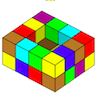Painting Possibilities

Age 7 to 11 Challenge Level:

This task, written for the National Young Mathematicians' Award 2016, involves open-topped boxes made with interlocking cubes. Explore the number of units of paint that are needed to cover the boxes. . . .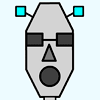Robot Monsters

Age 5 to 7 Challenge Level:

Use these head, body and leg pieces to make Robot Monsters which are different heights.Making Trains

Age 5 to 7 Challenge Level:

Can you make a train the same length as Laura's but using three differently coloured rods? Is there only one way of doing it?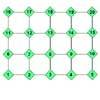Routes 1 and 5

Age 5 to 7 Challenge Level:

Find your way through the grid starting at 2 and following these operations. What number do you end on?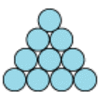Difference

Age 7 to 11 Challenge Level:

Place the numbers 1 to 10 in the circles so that each number is the difference between the two numbers just below it.Homes

Age 5 to 7 Challenge Level:

There are to be 6 homes built on a new development site. They could be semi-detached, detached or terraced houses. How many different combinations of these can you find?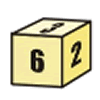First Connect Three for Two

Age 7 to 11 Challenge Level:

First Connect Three game for an adult and child. Use the dice numbers and either addition or subtraction to get three numbers in a straight line.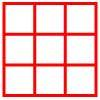Age 5 to 11 Challenge Level:

Place six toy ladybirds into the box so that there are two ladybirds in every column and every row.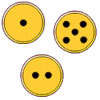Fair Exchange

Age 5 to 7 Challenge Level:

In your bank, you have three types of coins. The number of spots shows how much they are worth. Can you choose coins to exchange with the groups given to make the same total?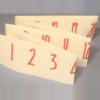Folded Number Line

Age 7 to 11 Challenge Level:

When I fold a 0-20 number line, I end up with 'stacks' of numbers on top of each other. These challenges involve varying the length of the number line and investigating the 'stack totals'.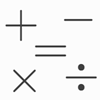Which Symbol?

Age 7 to 11 Challenge Level:

Choose a symbol to put into the number sentence.A Square of Numbers

Age 7 to 11 Challenge Level:

Can you put the numbers 1 to 8 into the circles so that the four calculations are correct?Code Breaker

Age 7 to 11 Challenge Level:

This problem is based on a code using two different prime numbers less than 10. You'll need to multiply them together and shift the alphabet forwards by the result. Can you decipher the code?Zargon Glasses

Age 7 to 11 Challenge Level:

Zumf makes spectacles for the residents of the planet Zargon, who have either 3 eyes or 4 eyes. How many lenses will Zumf need to make all the different orders for 9 families?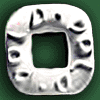Polo Square

Age 7 to 11 Challenge Level:

Arrange eight of the numbers between 1 and 9 in the Polo Square below so that each side adds to the same total.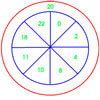On Target

Age 7 to 11 Challenge Level:

You have 5 darts and your target score is 44. How many different ways could you score 44?One Million to Seven

Age 7 to 11 Challenge Level:

Start by putting one million (1 000 000) into the display of your calculator. Can you reduce this to 7 using just the 7 key and add, subtract, multiply, divide and equals as many times as you like?Domino Numbers

Age 7 to 11 Challenge Level:

Can you see why 2 by 2 could be 5? Can you predict what 2 by 10 will be?Here to There 1 2 3

Age 5 to 7 Challenge Level:

Move from the START to the FINISH by moving across or down to the next square. Can you find a route to make these totals?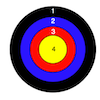Dart Target

Age 7 to 11 Challenge Level:

This task, written for the National Young Mathematicians' Award 2016, invites you to explore the different combinations of scores that you might get on these dart boards.Arranging the Tables

Age 7 to 11 Challenge Level:

There are 44 people coming to a dinner party. There are 15 square tables that seat 4 people. Find a way to seat the 44 people using all 15 tables, with no empty places.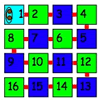Caterpillars

Age 5 to 7 Challenge Level:

These caterpillars have 16 parts. What different shapes do they make if each part lies in the small squares of a 4 by 4 square?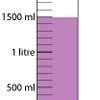Oh! Harry!

Age 7 to 11 Challenge Level:

A group of children are using measuring cylinders but they lose the labels. Can you help relabel them?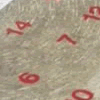All Seated

Age 7 to 11 Challenge Level:

Look carefully at the numbers. What do you notice? Can you make another square using the numbers 1 to 16, that displays the same properties?A-magical Number Maze

Age 7 to 11 Challenge Level:

This magic square has operations written in it, to make it into a maze. Start wherever you like, go through every cell and go out a total of 15!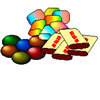The Puzzling Sweet Shop

Age 7 to 11 Challenge Level:

There were chews for 2p, mini eggs for 3p, Chocko bars for 5p and lollypops for 7p in the sweet shop. What could each of the children buy with their money?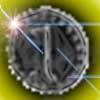Mrs Beeswax

Age 5 to 7 Challenge Level:

In how many ways could Mrs Beeswax put ten coins into her three puddings so that each pudding ended up with at least two coins?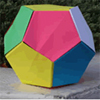Dodecamagic

Age 7 to 11 Challenge Level:

Here you see the front and back views of a dodecahedron. Each vertex has been numbered so that the numbers around each pentagonal face add up to 65. Can you find all the missing numbers?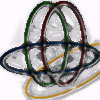Bean Bags for Bernard's Bag

Age 7 to 11 Challenge Level:

How could you put eight beanbags in the hoops so that there are four in the blue hoop, five in the red and six in the yellow? Can you find all the ways of doing this?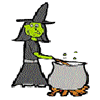Hubble, Bubble

Age 7 to 11 Challenge Level:

Winifred Wytsh bought a box each of jelly babies, milk jelly bears, yellow jelly bees and jelly belly beans. In how many different ways could she make a jolly jelly feast with 32 legs?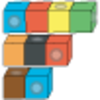Making Longer, Making Shorter

Age 5 to 7 Challenge Level:

Ahmed is making rods using different numbers of cubes. Which rod is twice the length of his first rod?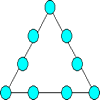Magic Triangle

Age 7 to 11 Challenge Level:

Place the digits 1 to 9 into the circles so that each side of the triangle adds to the same total.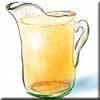Pouring the Punch Drink

Age 7 to 11 Challenge Level:

There are 4 jugs which hold 9 litres, 7 litres, 4 litres and 2 litres. Find a way to pour 9 litres of drink from one jug to another until you are left with exactly 3 litres in three of the jugs.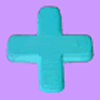Age 7 to 11 Challenge Level:

Can you put plus signs in so this is true? 1 2 3 4 5 6 7 8 9 = 99 How many ways can you do it?The Pied Piper of Hamelin

Age 7 to 11 Challenge Level:

This problem is based on the story of the Pied Piper of Hamelin. Investigate the different numbers of people and rats there could have been if you know how many legs there are altogether!Pass the Peas, Please

Age 5 to 7 Challenge Level:

A game for 2 or more players. Practise your addition and subtraction with the aid of a game board and some dried peas!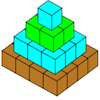Open Squares

Age 7 to 11 Challenge Level:

This task, written for the National Young Mathematicians' Award 2016, focuses on 'open squares'. What would the next five open squares look like?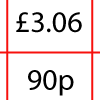How Much Did it Cost?

Age 7 to 11 Challenge Level:

Use your logical-thinking skills to deduce how much Dan's crisps and ice-cream cost altogether.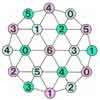Totality

Age 5 to 11 Challenge Level:

This is an adding game for two players.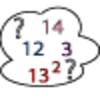Seven Square Numbers

Age 7 to 11 Challenge Level:

Add the sum of the squares of four numbers between 10 and 20 to the sum of the squares of three numbers less than 6 to make the square of another, larger, number.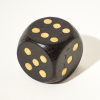Double or Halve?

Age 5 to 7 Challenge Level:

Throw the dice and decide whether to double or halve the number. Will you be the first to reach the target?Rabbits in the Pen

Age 7 to 11 Challenge Level:

Using the statements, can you work out how many of each type of rabbit there are in these pens?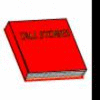Page Numbers

Age 7 to 11 Short Challenge Level:

Exactly 195 digits have been used to number the pages in a book. How many pages does the book have?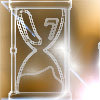Two Egg Timers

Age 7 to 11 Challenge Level:

You have two egg timers. One takes 4 minutes exactly to empty and the other takes 7 minutes. What times in whole minutes can you measure and how?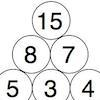Build it up More

Age 7 to 11 Challenge Level:

This task follows on from Build it Up and takes the ideas into three dimensions!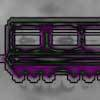Train Carriages

Age 5 to 11 Challenge Level:

Suppose there is a train with 24 carriages which are going to be put together to make up some new trains. Can you find all the ways that this can be done?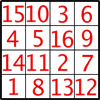Magic Constants

Age 7 to 11 Challenge Level:

In a Magic Square all the rows, columns and diagonals add to the 'Magic Constant'. How would you change the magic constant of this square?Sums and Differences 2

Age 7 to 11 Challenge Level:

Find the sum and difference between a pair of two-digit numbers. Now find the sum and difference between the sum and difference! What happens?Sums and Differences 1

Age 7 to 11 Challenge Level:

This challenge focuses on finding the sum and difference of pairs of two-digit numbers.Money Line-up

Age 5 to 7 Challenge Level:

In this game for two players, the aim is to make a row of four coins which total one dollar.